1. /
2. CBSE
3. /
4. Class 08
5. /
6. Mathematics
7. /
8. NCERT Solutions for Class...

# NCERT Solutions for Class 8 Maths Exercise 4.4### myCBSEguide App

Download the app to get CBSE Sample Papers 2023-24, NCERT Solutions (Revised), Most Important Questions, Previous Year Question Bank, Mock Tests, and Detailed Notes.

NCERT solutions for Class 8 Maths Practical Geometry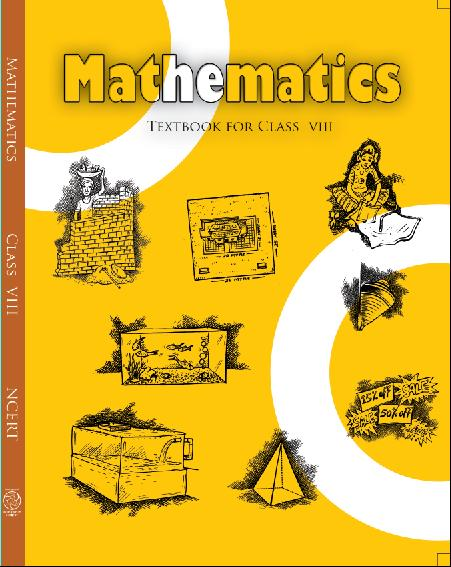## NCERT Solutions for Class 8 Maths Practical Geometry

###### 1. Construct the following quadrilaterals:

DE = 4 cm, EA = 5 cm, AR = 4.5 cm,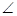E =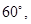A =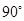TR = 3.5 cm, RU = 3 cm, UE = 4 cm,R =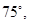U =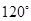Ans.

(i) Given:  DE = 4 cm, EA = 5 cm,

AR = 4.5 cm,E =A =NCERT Solutions for Class 8 Maths Exercise 4.4

###### Steps of construction: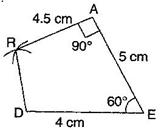(a) Draw a line segment DE = 4 cm.

(b) At point E, construct an angle of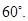(c) Taking radius 5 cm, draw an arc from point E which intersects at A.

(d) ConstructA =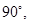draw an arc of radius 4.5 cm with centre A which intersect at R.

(e) Join RD.

It is the required quadrilateral DEAR.

(ii) Given:  TR = 3.5 cm, RU = 3 cm,

UE = 4 cm,R =U =NCERT Solutions for Class 8 Maths Exercise 4.4

###### Steps of construction: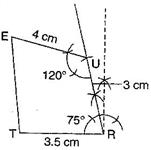(a) Draw a line segment TR = 3.5 cm.

(b) Construct an angle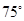at R and draw an arc of radius 3 cm with R as centre, which intersects at U.

(c) Construct an angle ofat U and produce the side UE.

(d) Draw an arc of radius 4 cm with U as centre.

(e) Join UE and TE.

It is the required quadrilateral TRUE.

## NCERT Solutions for Class 8 Maths Exercise 4.4

NCERT Solutions Class 8 Mathematics PDF (Download) Free from myCBSEguide app and myCBSEguide website. Ncert solution class 8 Mathematics includes text book solutions from Class 8 Maths Book . NCERT Solutions for CBSE Class 8 Maths have total 16 chapters. 8 Maths NCERT Solutions in PDF for free Download on our website. Ncert class 8 solutions PDF and Maths ncert class 8 PDF solutions with latest modifications and as per the latest CBSE syllabus are only available in myCBSEguide.

## CBSE app for Class 8

To download NCERT Solutions for class 8 Social Science, Computer Science, Home Science,Hindi ,English, Maths Science do check myCBSEguide app or website. myCBSEguide provides sample papers with solution, test papers for chapter-wise practice, NCERT solutions, NCERT Exemplar solutions, quick revision notes for ready reference, CBSE guess papers and CBSE important question papers. Sample Paper all are made available through the best app for CBSE students and myCBSEguide website.Test Generator

Create question paper PDF and online tests with your own name & logo in minutes.myCBSEguide

Question Bank, Mock Tests, Exam Papers, NCERT Solutions, Sample Papers, Notes

### 2 thoughts on “NCERT Solutions for Class 8 Maths Exercise 4.4”

1. This app is best

2. this is good way